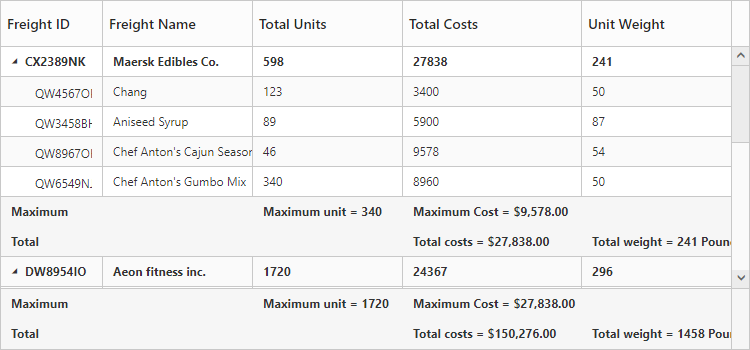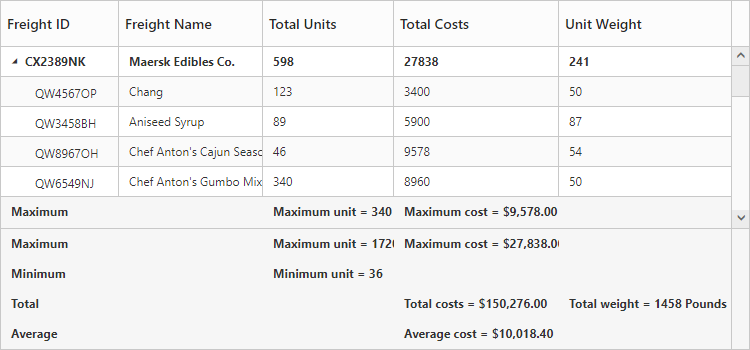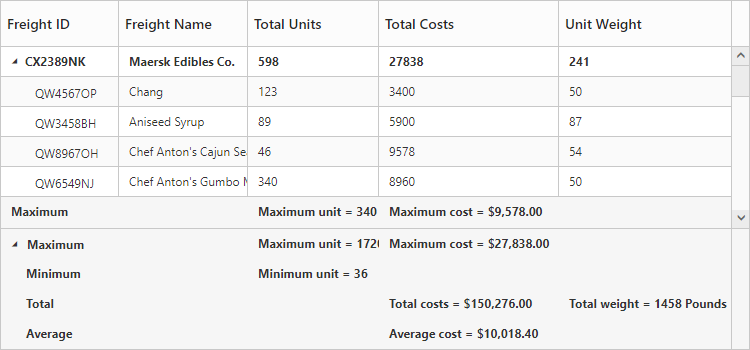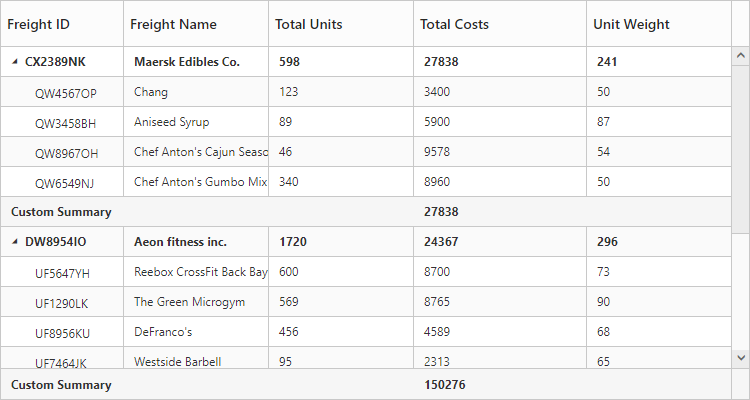# Summary Row

24 Sep 20187 minutes to read

Summary rows in TreeGrid is used to summarize every hierarchy with the set of predefined summary types using the column values.

• `SummaryRows` - Using this property, user can define the summary rows in TreeGrid.
• `SummaryRows.Title` - Title for each summary row can be defined using this property.
• `SummaryRows.SummaryColumns` - Using this property, it is possible to defined the summary for specific columns alone in a summary row.
• `ShowSummaryRow` - This property is to make the summary row visible.
• `ShowTotalSummary` - This property is to make the total summary row visible which is the overall summary row displayed for all the rows in the TreeGrid content.

## Defining summary columns

• `SummaryType` - Using this property, user can define the type of summary to be displayed in a column.
• `DataMember`- This property is used to map the field values which is used for summary calculations.
• `DisplayColumn`- This property is used to specify the column in which the summary to be displayed.
• `Prefix` and `Suffix` properties are used to define the text should be displayed along with the summary column value.
• `Format`- property is used for formatting the summary column value.

The below code snippet explains defining a summary row in TreeGrid,

• HTML
• ``````<ej:TreeGrid runat="server" ShowSummaryRow="true" ShowTotalSummary="true" ID="TreeGridControlSummaryRow">
<SummaryRows>
<ej:TreeGridSummaryRow Title="Maximum">
<SummaryColumn>
<ej:TreeGridSummaryColumn SummaryType="Maximum" DataMember="TotalUnits" DisplayColumn="TotalUnits"  Prefix="Maximum unit = "/>
</SummaryColumn>
<SummaryColumn>
<ej:TreeGridSummaryColumn SummaryType="Maximum" DataMember="TotalCosts" DisplayColumn="TotalCosts" Prefix="Maximum Cost = " Format="{0:C}" />
</SummaryColumn>
</ej:TreeGridSummaryRow>
<ej:TreeGridSummaryRow Title="Total">
<SummaryColumn>
<ej:TreeGridSummaryColumn SummaryType="Sum" DataMember="TotalCosts" DisplayColumn="TotalCosts" Prefix="Total costs =  " Format= "{0:C}" />
<ej:TreeGridSummaryColumn SummaryType="Sum" DataMember="UnitWeight" DisplayColumn="UnitWeight" Prefix="Total weight = " Suffix=" Pounds" />
</SummaryColumn>
</ej:TreeGridSummaryRow>
</SummaryRows>
</ej:TreeGrid>``````

The below screenshot shows the output of above code example.## Customize height of total summary

Using `TotalSummaryHeight` property we can customize the height of the total summary container.
The below code example shows how to update the footer summary container height.

• HTML
• ``````<ej:TreeGrid runat="server" ID="TreeGridControlSummaryRow"
ShowSummaryRow="true"
ShowTotalSummary="true"
TotalSummaryHeight="120">
//..
</ej:TreeGrid>``````

The below screenshot shows the output of above code example.## Expand/collapse total summary row

We can expand/collapse the total summary rows in TreeGrid using following methods.

• Using Expander Icon
• Using Method

### Using Expander Icon

We can enable expander icon in total summary row by using `CollapsibleTotalSummary` property. By default expander icon will be rendered in first row of 0th column in total summary rows.
Please find the below code example to enable collapsible total summary row in TreeGrid.

• HTML
• ``````<ej:TreeGrid runat="server" ID="TreeGridControlSummaryRow"
ShowSummaryRow="true"
ShowTotalSummary="true"
TotalSummaryHeight="120"
CollapsibleTotalSummary="true" >
//..
</ej:TreeGrid>``````NOTE

We can also customize the expander icon column in total summary row by using `_summaryColumnIndex` property and `Load` event.

### Using Method

Total summary rows in TreeGrid can be expanded/collapsed by using `expandCollapseTotalSummary` method.
Please find the code example to collapse the total summary rows below.

• HTML
• ``````<button onclick="expandCollapse()">expandCollapse</button>

<ej:TreeGrid runat="server" ID="TreeGridControlSummaryRow"
ShowSummaryRow="true"
ShowTotalSummary="true"
TotalSummaryHeight="120"
CollapsibleTotalSummary="true" >
//..
</ej:TreeGrid>
function expandCollapse() {
var treeObj = \$("#TreeGridContainer").data("ejTreeGrid");
treeObj.expandCollapseTotalSummary(false);
}``````

Click here to view the demo sample for summary row in TreeGrid.

## Custom Summary

Custom summary can be used to create summary values based on your required custom logic and calculations. To enable the custom summary, the `SummaryType` should be set to ‘Custom’ and the `CustomSummaryValue` property should be defined as function. After the custom calculation, the returned value will be displayed in the corresponding summary cell.

• HTML
• ``````<ej:TreeGrid runat="server" ID="TreeGridSummary" ShowSummaryRow="true">
<SummaryRows>
<ej:TreeGridSummaryRow Title="Custom Summary" >
<SummaryColumn>
<ej:TreeGridSummaryColumn SummaryType="Custom" CustomSummaryValue="sum" DisplayColumn="TotalCosts" />
</SummaryColumn>
</ej:TreeGridSummaryRow>
</SummaryRows>
</ej:TreeGrid>
</div>
</form>``````
• JS
• ``````<script>
function sum(args, data) {
//ej.sum is aggregate to add data of total costs from datasource
return ej.sum(data, "TotalCosts");
}
</script>``````

The output of the tree grid with custom summary value is as follows.